# Difference between revisions of "Tait equation of state"

The Tait equation is an equation of state. The equation was originally published by Peter Guthrie Tait in 1888 . It may be written as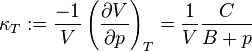$\kappa_T := \frac{-1}{V} \left ( \frac{\partial V}{\partial p} \right )_T = \frac{1}{V} \frac{C}{B+p}$

or in the integrated form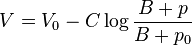$V = V_0 - C \log \frac{B+p}{B+p_0}$

where

•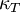$\kappa_T$ is the Isothermal compressibility
•$V \$ is the specific volume.
•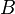$B \$ and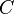$C \$ are functions of temperature that are independent of pressure.

It is quite common that this name is improperly used for the adiabatic form of the stiffened equation of state.